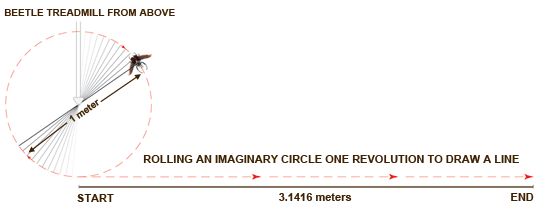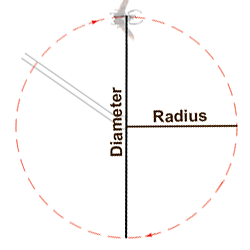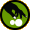# Dr. Biology Visits with Biologist Jon Harrison

Dr. Biology pops into the laboratory of biologist Jon Harrison to see what's happening with his giant beetles. It's not long before he gets a lesson on how insects breathe differently than humans. He also takes a quick journey back 300 million years - when these crawling and flying animals were super-sized. You can see one of these modern day creatures exercising on a cool beetle flightmill. It is kind of a treadmill for flying animals. Below we have the story on the red light and also the math used to calculate how far and fast these beetles fly.

The Red LightYou might think the red light in the video was added to make the lab look more scientific. It's really a normal feature of the room. As part of the fire safety code, a light needs to be left on in the room at all times. The beetles are also kept in the room and need a normal light cycle where they have a dark period. The answer was to place a red bulb in the safety light. Most insects including the beetles cannot see red light. This allows them to have a period of time during each day when the white lights are off and for the beetles dark.

Beetle Flightmill Math

How can you figure out how far and how fast a beetle flies on their custom treadmill? There are two ways. One would be to draw out a line of the imaginary circle the beetle flies.The other way is to use mathematics. This is much easier and more practical than dealing with imaginary circles. To setup our calculation we need to learn about Pi (pie). Not the pie you eat, but the mathematical constant that can be used to figure out the circumference of a circle. It is also used for other calculations, but for this activity we will be figuring out the diameter of the imaginary circle the beetle flies. After we know the circumference of the circle we can find out how far and how fast the beetle travels.Pi (Pie for math)

We call Pi a constant because it represents the ratio of a circle's circumference to its diameter. It is also an irrational number. This means it can be calculated out to an infinite number of decimals and never repeats.

Pi goes forever - 3.141592653589793238462643383279502884197169399375...For our calculation we need to know the diameter of the circle. This is the length of the flight bar and the value of Pi. The flight bar is 1 meter. The calculation follows.

1 * 3.1416 = 3.1416 meters

Now we know distance the beetle flies with each revolution (3.1416 meters). We also know it takes the beetle one second to make each revolution.  We can now calculate speed.

• In the video the beetle flies about one revolution per second. Thus its speed is 3.1416 meters per second.
• Multiply by 60 and we have 188.5 meters per minute.
• Multiply by 60 again and we have 11309.7 meters per hour
• Divide by 1000 and we have 11.3 kilometers per hour.
• Divide that by 1.6 and we get 7.07 miles per hour.

For distance we total the number of revolutions.

• Imagine the beetle flies at a constant speed for exactly 5 minutes.
• 5 times 60 is 300 seconds.
• 300 times 3.1416 meters gives a distance of 942.48 meters.
• Divide by 1000 and we have 0.942 kilometers.
• Divide by 1.6 and we get a distance of 0.59 miles.

Now you try it

• The fastest flying beetle we have had can complete a revolution every .75 seconds. How far does it fly in 5 minutes?
• The longest time we have had a beetle fly continuous is 17 minutes. How far did it travel if it completed each revolution in 1 second?

Thanks to Dr. C. Jaco Klok for sharing the beetle mathematics activity.You can read more about Jon Harrison and his research with insects in the companion article - The Interest of Insects.

## Be Part of Ask A Biologist

By volunteering, or simply sending us feedback on the site. Scientists, teachers, writers, illustrators, and translators are all important to the program. If you are interested in helping with the website we have a Volunteers page to get the process started.Contribute